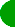Go to ECE394   Experiment   |  1  |   2   |   3   |   4   |   6   |  7   |  8   |  9   |      Lab manuals  |   ECE Lab home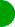ECE394 - Digital Systems Laboratory

Experiment

Shift Registers

1.  Introduction

A shift register is an n-bit register with provision for shifting its stored data by one position at each clock pulse.  The logical configuration of a shift register consists of a chain of flip-flops connected in cascade, with the output of one flip-flop connected to the input of the next flip-flop.  All flip-flops receive a common clock pulse which causes the shift from one stage to the next.  Fig. 1 shows a simple shift register configuration.  The new bit to be shifted into one end must be specified, and the bit shifted off the other end is lost unless it is saved externally.  Although Fig. 1 shows a right-shift register, the same register can obviously be used for left shifts simply by reversing the sense of the bits.  Most shift registers have provision for shifting only in one direction, but some have a control input that allows either left or right shifting to be specified at each clock.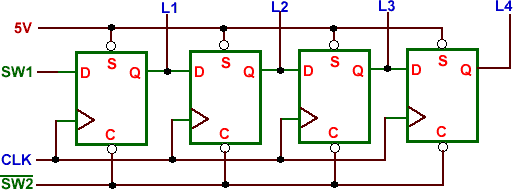Fig. 1. 4-bit shift register.

One way to load n bits of data into the flip-flop chain is to load the data one bit each clock cycle using the serial input.  Some shift registers also have parallel inputs that can be used to load all n bits in one clock cycle.  The output of a shift register can be observed one bit at a time at the serial output, but some shift registers also have parallel outputs for observing all n bits at once.

Shift registers are classified according to three basic considerations: their method of data handling (serial-in serial-out, serial-in parallel-out, and parallel-in serial-out), their direction of data movement (shift right, shift left, and bidirectional), and their bit length.  One of the important applications of shift register circuits is in serial computation.  Compared to parallel computation, where all bits in a word are processed at the same cycle, serial computation process words in one bit per cycle.  Therefore, serial computation is slower, but it has the advantage of requiring less hardware and wiring.  A serial adder will be build in this experiment as an example.

2.   Objectives

Students are expected to understand various data handling methods in shift registers and their usage.

3.   Experiment

3.1.  4-bit Shift Register

Use two 7474 dual flip-flops to connect a serial-in, parallel-out shift register as shown in Fig. 1.  Connect L1–L4 to four LEDs, SW1 and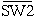to switches, and CLK to a pulser. Initially, setto logic 1.  Switchbeing at logic 1 clears all flip-flops.  Now set SW1 to logic 1 andto logic 0.  Push the pulser button several times to allow more logic 1 to be shifted into the shift register.  Change SW1 to logic 0 and repeat the experiment again.

3.2. Pseudo-random Sequence Generator

Now use the above circuit to build a pseudo-random binary sequence generator as shown in Fig. 2.  This binary sequence generator will display a random output (repeats every 2n–1 bits, where n is the number of flip-flops used in the shift register).  The IC 7486 provides the exclusive-OR needed in the circuit.  To start the sequence generator, set the initial state of the shift register to 0001 by setting the switch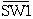to logic 1.  Then changeto logic 0 as this will release the control input.  Now apply the clock and record the output in a table.  Does the output show randomness?  Does the output repeat after 15 pulses?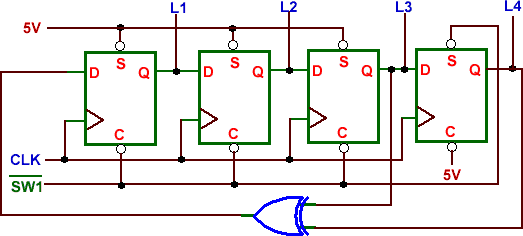Fig. 2. Pseudo-random binary sequence generator.

A serial adder adds bits adds a pair of binary numbers serially with a simple full adder.  The carry out of the full adder is transferred into a D flip-flop and the output of this carry flip-flop is then used as the input carry for the next pair of significant bits.  Fig. 3 illustrates an example of serial addition.

 ...1 0 1 1 1 0  ...1 1 1 0 1 1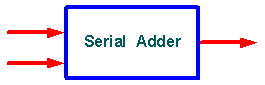...1 0 1 0 0 1 Fig. 3. Serial addition.

Fig. 4. shows the block diagram design of a serial adder.  The two binary numbers to be added serially are stored in two shift registers (using two 74164, 8-bit serial-in, parallel-out shift registers).  Bits are added one pair at a time through a single full adder (such as the one in Experiment 4)  The carry out of the full adder is transferred into a D flip-flop.  The output of this carry flip-flop is then used as the input carry for the next pair of significant bits.  The sum output from the full adder is transferred into register A as the contents of the register are shifted out.

To perform the addition, the following steps are used.

1. Shift the first 8-bit augend into A (remember to shift in LSB first) and addend into B.  This is done by

(i) shifting the augend through the serial input;

(ii) clearing A and the D flip-flop;

(iii) shift the addend through the serial input;

2. Clear the D flip-flop and run the adder for eight cycles to obtain the sum.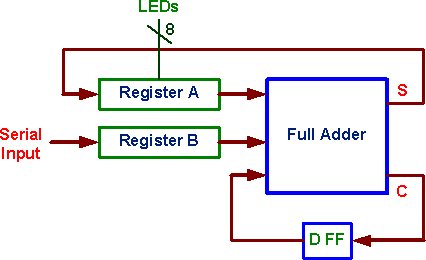Fig. 4. Block diagram of a serial adder.

4.   Prelab

1. Study the operation of the 74164.

2. Draw a  circuit diagram of the serial adder showing the connections for all the components.

3. What is the maximum clock speed for the adder circuit of part 3.3? Assume worst case delays and 74LSXX type logic.

5.  Equipment and parts required.

• Protoboard

• Two TTL D Flip-Flops (7474)

• One TTL NAND (7400)

• One TTL inverter (7404)

• One TTL XOR (7486)

• Two TTL Shift registers (74164)

• Eight LEDs and limiting resistorsGo to ECE394   Experiment   |  1  |   2   |   3   |  4  |   6   |  7   |  8   |  9   |      Lab manuals  |   ECE Lab home